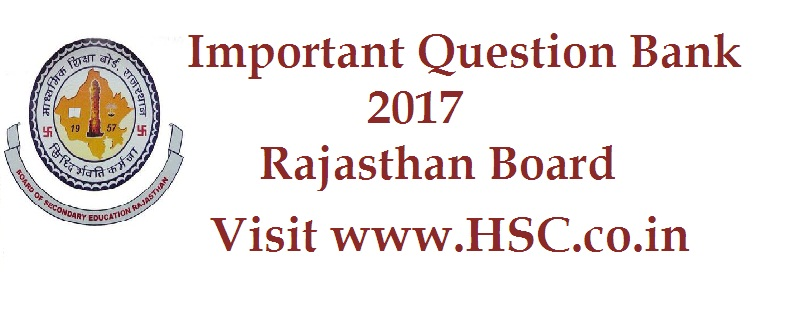# Maths – Important Question Bank for Rajasthan class 12th (XII) (HSC) Board Exam 2017

HSC Board Exams are fast approaching and students are getting anxious about how to prepare for their HSC Board Exams. So we had mentioned some

After the tremendous success of our last year Important Question Bank for Rajasthan class 12 (HSC) Board Exam 2016, we have also created a list of  Most Important Question Bank for Rajasthan class 12th (XII) (HSC) Board Exam 2017 which are likely to appear in HSC Board Exams this year.To unlock the content Click on any of 3 buttons available in the box below saying “This content is Locked”. Once you click on the button the content will get unlocked on same page itself. You must click on social media button showing in below box ie Facebook, Twitter or Google Plus to unlock the content.

Hi, we’re trying to collate and gather the data and would be updating it here a few days before the exam. Please keep on visiting our website for updates.

Please use the comments box below and post questions that you think are important from your analysis. It would help the HSC community a lot.

Do subscribe to our updates so that you do not miss out on any important information that we push your way.

1. From a lot of 30 bulbs which include 6 defectives, a sample of 2 bulbs is drawn at random with replacement. Find the probability distribution of the number of defective bulbs

2. Bag A contains 2 red and 3 black balls while another bag B contains 3 red and 4 black balls. One ball is drawn at random from one of the bag and it is found to be red. Find the probability that it was drawn from bag B.

3. By graphical method solve the following linear programming problem for maximization

Objective function Z = 1000x to 600y

Constraints x + y ≤ 200

4x – y ≤ 0

X ≥ 20, x ≥ 0, y ≥ 0.

4. Using integration find the area of a triangular region whose sides have the equations y = x + 1, y = 2x + 1 and x = 2. (draw the figure in answer book)

5. Find the area bounded by the parabola y2 = 4x and the straight line y = x. (draw the figure in answer book)

6. The radius of a circle is increasing uniformly at the rate of 5cm/sec. find rate at which the area of the circle is increasing when the radius is 6cm.

7. Find the intervals in which the function f given by f(x) = x2 – 6x +5 is

Strictly increasing

Strictly decreasing

8. Prove that the relation R in set of real numbers R defined as R = {(a, b): a ≥ b} is reflexive and transitive but not symmetric

9. Consider f: R → R given by f(x) = 2x + 3. Show that f is invertible. Find also the inverse of function f.

10. If A and B are independent events with P(A) = 0.2 and P(B) = 0.5, then find the value of P(A ∪ B)

11. Show the region of feasible solution under the following constraints 2x + y ≥ 8, x ≥ 0 y ≥ 0 in answer book.

12.  Find the equation of the tangent to the curve x 2/3 + y 2/3 = 1 at the point (1, 1)

Don’t forget to read : MUST REMEMBER THINGS on the day of Exam for HSC Students